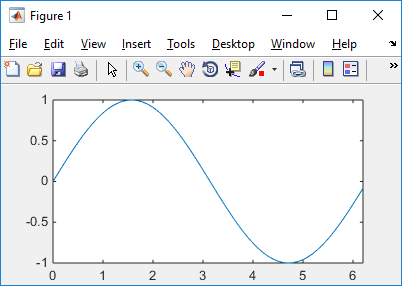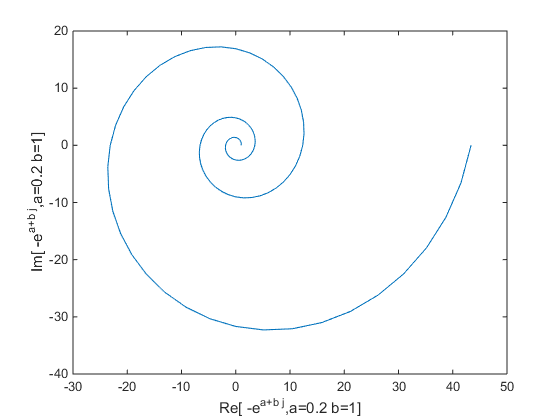Matlab - Short Tips                                                      Home : www.sharetechnote.com

Followings are list of the short tips that may be useful for you.

set(gcf, 'Position', [startX startY width height])

Example 01 >

 Ex 1 >  This has been tested in Matlab only (not in Octave) t = 0:0.1:2*pi; plot(t,sin(t)); xlim([min(t) max(t)]); set(gcf, 'Position', [100 100 400 200]); OutputExample 01 >

 Ex 1 >  This has been tested in Matlab only (not in Octave) t=0:2*pi/40:6*pi; a = 0.2; b = 1.0; f = exp((a+b*j)*t);   plot(real(f),imag(f)); xlabel(strcat('Re[ -e^{a+b j},a=',num2str(a),' b=',num2str(b),']')); ylabel(strcat('Im[ -e^{a+b j},a=',num2str(a),' b=',num2str(b),']')); OutputDeleting All Text in Graph

t = findall(gcf,'type','text');

delete(t);

Changing Background Color in Graph

set(gcf,'color','w');

Flatten 2D Matrix to 1D Vector

matA = reshape(matA ,[] ,1) % Flatten to a Column Vector

matA = reshape(matA ,1, []) % Flatten to a Row Vector

imshow(img,'InitialMagnification','fit');